# 21 Practice Exercises

## Multiple Choice Questions

Exercise: Stoichiometry

Which of the following statements is true about the reaction $A+B \rightarrow P$?

a) the stoichiometric coefficient for A is 1

b) the stoichiometric coefficient for B is -1

c) the reaction extent can be positive, negative

d) the stoichiometric coefficient for P is -1

### Solution

b) the stoichiometric coefficient for B is -1
The stoichiometric coefficient is negative for reactants and positive for products. Since the reaction only goes forward, the reaction extent is always positive (or 0 if it doesn’t happen)

Exercise: Stoichiometric Expression

Which of the following statement is true about the equilibrium reaction $A+B \leftrightarrow P$?

a) the stoichiometric coefficient for A is 1

b) the stoichiometric coefficient for P is -1

c) the reaction extent can be positive or negative

d) the equilibrium constant K can be positive or negative

### Solution

c) the reaction extent can be positive, negative

The stoichiometric coefficient is negative for reactants and positive for products. Since it is an equilibrium reaction, if the reverse reaction happens more frequently than the forward reaction, the reaction extent can be negative. The equilibrium constant is $K=\prod_{i}a_{i,eq}^{vi}$. Since the concentration of any compound must be positive, the equilibrium constant K must be positive as well.

Exercise: Reaction Order

The following data shows the concentration of reactive species [A] in the system at points of time.

 Time (s) [A] (mol/L) 0 2.000 0.100 0.286 0.200 0.154 0.300 0.105

What is the reaction order of A, determined by the integrated rate law?

a) zeroth-order

b) first-order

c) second-order

d) none of the above

### Solution

c) second-order

Zeroth order: $[A]-[A]_{0}=-k_{r}×t$, would be linear if graph $[A]$ against $t$
First order: $ln[A]-ln[A]_{0}=-k_{r}*t$, would be linear if graph $ln([A])$ against $t$
Second order: $\frac{1}{[A]}-\frac{1}{[A]_{0}}=k_{r}*t$, would be linear if graph $\frac{1}{[A]}$ against $t$So we plot $[A]$ against $t$, $ln([A])$ against $t$, and $\frac{1}{[A]}$ against $t$ and see which one is linear: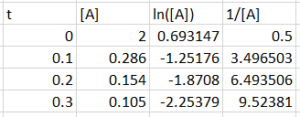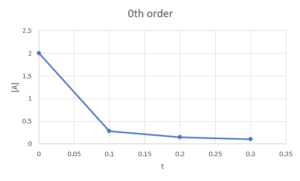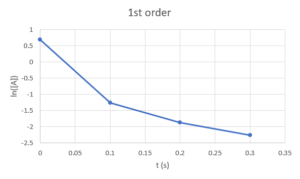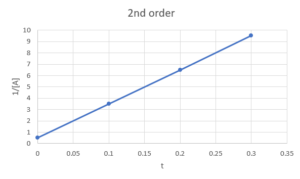We can see that only $\frac{1}{[A]}$ against $t$  is linear, so the reaction is second order in A.

Exercise: Reaction Rate Law Units

$H_2O_{2(aq)} + 3 I^-_{(aq)} + 2H^+_{(aq)}\rightarrow I^-_{3(aq)} +H_2O_{(l)}$

For this reaction, the reaction rate law expression is $r=k_r[H_2O_2][I^-]$.

If the concentration of $[H_2O_2]$, $[I^-]$ and $[H^+]$ are measured in units of mol/L, what is the unit of the reaction rate constant $k_r$?

a) $\frac{L^2}{mol^2L}$

b)$\frac{mol}{L·s}$

c) $\frac{L}{mol·s}$

d) $\frac{L^5}{mol^5·s}$

### Solution

c) $\frac{L}{mol·s}$

Based on the unit that the reactive species are measured, we can know that the unit for $r$ is $\frac{mol}{L·s}$. So we can equate the units on both sides of the rate law:
\begin{align*}
\frac{mol}{L·s}&=k_r×\frac{mol}{L}×\frac{mol}{L}\\
\frac{mol}{L·s}×\frac{L}{mol}×\frac{L}{mol}&=k_r\\
\frac{L}{mol·s}&=k_r
\end{align*}

Exercies: Reaction Rates

We have the following reaction happening in a fixed reaction chamber:

$2NH_{3(g)} \rightarrow N_{2(g)} +3H_{2(g)}$

The partial pressure of $NH_3$ was measured at each time point and shown in the table below.

 Time (ms) $[NH_{3}]$ (Pa) 0 1670 100 1300 200 1012 300 789 400 614 500 478

a) Calculate the initial rate of reaction

b) Calculate the initial rate of change in partial pressure for every compound involved in the reaction

*Hint: for small time intervals, we can take $\frac{d[J]}{dt}=\frac{\Delta[J]}{\Delta t}$, where J is any species involved in the reaction

### Solution

a) \begin{align*}
r&=\frac{1}{v_j}\frac{d[J]}{dt}=\frac{1}{v_j}\frac{\Delta[J]}{\Delta t}\\
&=\frac{1}{-2}\frac{\Delta[NH_{3}]}{\Delta t}\\
&=\frac{1}{-2}\frac{(1300-1670)Pa}{(100-0)ms×\frac{1s}{1000ms}}\\
&=1850 Pa/s
\end{align*}

b) Because is it a “fixed reaction chamber”, which means the total volumn of the gas chamber stays constant, so we can do the following balance using the definition of reaction rate:

$r=\frac{1}{-2}\frac{\Delta[NH_3]}{\Delta t}=\frac{\Delta[N_2]}{\Delta t}=\frac{1}{3}\frac{\Delta[H_2]}{\Delta t}$

$\frac{\Delta[NH_3]}{\Delta t}=-2×r=-2×1850Pa/s=-3700Pa/s$

$\frac{\Delta[N_2]}{\Delta t}=r=1850Pa/s$

$\frac{\Delta[H_2]}{\Delta t}=3×r=5550Pa/s$

Exercise: Reaction Order and Rate Constant

$^{}$ At high temperatures, nitrogen dioxide decomposes to nitric oxide and oxygen.

$2NO_{2(g)}→2NO_{(g)}+O_{2(g)}$

Experimental data for the reaction at 300°C and four initial concentrations of NO2 are listed in the following table:

 Experiment $[NO_{2}]_{0}$(M) Initial rate(M/s) 1 0.015 $1.22×10^{-4}$ 2 0.010 $5.40×10^{-5}$ 3 0.0080 $3.46×10^{-5}$ 4 0.0050 $1.35×10^{-5}$

### Solution

Step 1: Determine the reaction order with respect to nitrogen dioxide

For example, comparing Experiments 2 and 4 shows that doubling the concentration quadruples the reaction rate

$(5.40 × 10^{−5}) ÷ (1.35 × 10^{−5}) = 4.0$, which means that the reaction rate is proportional to $[NO_{2}]^2$.

Similarly, comparing Experiments 1 and 4 shows that tripling the concentration increases the reaction rate by a factor of 9, again indicating that the reaction rate is proportional to $[NO_{2}]^2$.

Therefore, the reaction is second order with respect to nitrogen dioxide.

Step 2: calculate $k_{r}$

We have $rate = k_{r}[NO_{2}]^2$. We can calculate the rate constant ($k_{r}$) using data from any experiment in the table.

\begin{align*}
rate & = k_{r}[NO_{2}]^2 \\
5.40×10^{-5} M/s& = k_{r}×(0.010M)^2\\
0.54M^{-1}s^{-1} & = k_{r}
\end{align*}

Method 2

The following graph is produced when plotting $log(r_{0})$ vs $log([A_{0}])$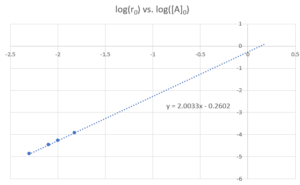From the line of best fit, we can see $a=2$, $log(k_{r})=-0.2602$

Therefore the reaction is first order in $NO_{2}$, $k_{r}=10^{-0.2602} = 0.55 M^{-1}s^{-1}$.

Exercise: Rate Constant and Arrhenius Equation

Suppose a reaction has an activation energy of 85 kJ/mol. If the reaction has a reaction rate constant of $70s^{-1}$ at 0°C, then what is the reaction rate constant at 25°C?

### Solution

We can use the rearranged Arrhenius Equation to relate the reaction rate constant to temperature. Note that the temperature should be expressed in K.
\begin{align*}
ln(\frac{k_{r2}}{k_{r1}})&=\frac{E_{a}}{R}\big(\frac{1}{T_{1}}-\frac{1}{T_{2}}\big)\\
ln\bigg(\frac{k_{r2}}{70s^{-1}}\bigg)&=\frac{85×10^3J/mol}{8.314J/(mol·K)}\bigg(\frac{1}{(0+273.15)K}-\frac{1}{(25+273.15)K}\bigg)\\
ln\bigg(\frac{k_{r2}}{70s^{-1}}\bigg)&=3.138\\
\frac{k_{r2}}{70s^{-1}}&=e^{3.138}\\
k_{r2}&=e^{3.138}×70s^{-1}\\
k_{r2}&=1615s^{-1}
\end{align*}

Exercise: Using Steady-state Approximation to Find the Rate Law Expression

Suppose we have a system with the reaction:

$H_{2}+Br_{2}→2H\!Br$

The elementary reactions are as follows: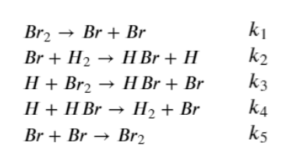Using the steady state assumption, we can prove that

$\frac{d[HBr]}{dt}=\frac{k_{a}[Br_{2}]^{3/2}[H_{2}]}{[Br_{2}]+k_{b}[H\!Br]}$

This will also allow us to determine the values of $k_{a}$ and $k_{b}$ in terms of the elementary reaction rate constants $k_{1}$ to $k_{5}$

### Solution

Step 1: Start by expressing the rate of change of the intermediates $Br$ and $H$, and set them equal to 0:

$\frac{d[Br]}{dt}=2k_{1}[Br_{2}]-k_{2}[Br][H_{2}]+k_{3}[H][Br_{2}]+k_{4}[H][H\!Br]-2k_{5}[Br]^2=0\;\;\;\;(1)$

$\frac{d[H]}{dt}=k_{2}[Br][H_{2}]-k_{3}[H][Br_{2}]-k_{4}[H][H\!Br]=0\;\;\;\;(2)$

Step 2: Express $\frac{d[HBr]}{dt}$ based on the elementary reactions:

$\frac{d[H\!Br]}{dt}=k_{2}[Br][H_{2}]+k_{3}[H][Br_{2}]-k_{4}[H][H\!Br]$

We can see that to get the espression in the question statement, we need to replace $[H]$ and $[Br]$ and simplify.

Step 3: Adding equations (1) and (2) together, we can see that 3 of the terms cancel, allowing us to solve for $[Br]$:
\begin{align*}
2k_{1}[Br_{2}]-2k_{5}[Br]^2 & = 0\\
2k_{1}[Br_{2}] & = 2k_{5}[Br]^2\\
(\frac{k_{1}}{k_{5}})^{1/2}[Br_{2}]^{1/2} & =[Br]
\end{align*}

Step 4: Next, we can determine $[H]$ using equation (2) by substituting what we derived for $[Br]$:
\begin{align*}
k_{2}[Br][H_{2}]-k_{3}[H][Br_{2}]-k_{4}[H][H\!Br] & = 0\\
(k_{3}[Br_{2}]+k_{4}[H\!Br])[H] & = k_{2}[Br][H_{2}]\\
[H] & =\frac{k_{2}[Br][H_{2}]}{k_{3}[Br_{2}]+k_{4}[H\!Br]}\\
[H] & = \frac{k_{2}(\frac{k_{1}}{k_{5}})^{1/2}[Br_{2}]^{1/2}[H_{2}]}{k_{3}[Br_{2}]+k_{4}[H\!Br]}
\end{align*}

Step 5: Substitute $[H]$ and $[Br]$ into $\frac{d[H\!Br]}{dt}$, then simplify
\begin{align*} \frac{d[HBr]}{dt} & = k_{2}[Br][H_{2}]+k_{3}[H][Br_{2}]-k_{4}[H][H\!Br]\\ & = k_{2}(\frac{k_{1}}{k_{5}})^{1/2}[Br_{2}]^{1/2}[H_{2}] +k_{3} \frac{k_{2}(\frac{k_{1}}{k_{5}})^{1/2}[Br_{2}]^{1/2}[H_{2}]}{k_{3}[Br_{2}]+k_{4}[H\!Br]} [Br_{2}]\\ & \;\;\;\;\;\;\;\;\;\;-k_{4}\frac{k_{2}(\frac{k_{1}}{k_{5}})^{1/2}[Br_{2}]^{1/2}[H_{2}]}{k_{3}[Br_{2}]+k_{4}[H\!Br]}[H\!Br]\\ & =k_{2}(\frac{k_{1}}{k_{5}})^{1/2}[Br_{2}]^{1/2}[H_{2}] *\\ & \;\;\;\;\;\;\;\;\;\; \Big[1+\frac{k_{3}[Br_{2}]}{k_{3}[Br_{2}]+k_{4}[H\!Br]}-\frac{k_{4}[HBr]}{k_{3}[Br_{2}]+k_{4}[H\!Br]}\Big]\\ & = k_{2}(\frac{k_{1}}{k_{5}})^{1/2}[Br_{2}]^{1/2}[H_{2}] *\\ & \;\;\;\;\;\; \;\;\;\;\Big[\frac{k_{3}[Br_{2}]+k_{4}[H\!Br]+k_{3}[Br_{2}]-k_{4}[H\!Br]}{k_{3}[Br_{2}]+k_{4}[H\!Br]}\Big]\\ & = k_{2}(\frac{k_{1}}{k_{5}})^{1/2}[Br_{2}]^{1/2}[H_{2}] * \frac{2k_{3}[Br_{2}]}{k_{3}[Br_{2}]+k_{4}[H\!Br]}\\ & = k_{2}(\frac{k_{1}}{k_{5}})^{1/2}[Br_{2}]^{1/2}[H_{2}] * \frac{2[Br_{2}]}{[Br_{2}]+\frac{k_{4}}{k_{3}}[H\!Br]}\\ &= \frac{2k_{2}(\frac{k_{1}}{k_{5}})^{1/2}[Br_{2}]^{3/2}[H_{2}]}{[Br_{2}]+\frac{k_{4}}{k_{3}}[H\!Br]} \end{align*}

Therefore, the values of $k_{a}$ and $k_{b}$ are:
$k_{a}=2k_{2}(\frac{k_{1}}{k_{5}})^{1/2}$

$k_{b}=\frac{k_{4}}{k_{3}}$

## Extra Reaction Mechanism Exercises

Reaction Mechanism Exercise 1

For the overall reaction

$2NO+2H_2 \rightarrow N_2 + 2H_2O$

The step-wise elementary reactions are: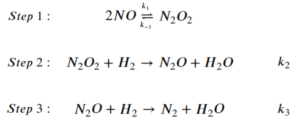Express reaction rate of the overall reaction using the steady-state approximation. The expression should only include $[NO]$, $[H_2]$, and the rate constants of the elementary steps.

### Solution

Step 1: Steady-state approximation states that the rate of generation and consumption of intermediates sum up to 0. The intermediates in the reaction are $N_2O_2$ and $N_2O$

\begin{align*}
&\frac{d[N_2O_2]}{dt}=k_1[NO]^2-k_{-1}[N_2O_2]-k_2[N_2O_2][H_2]=0\;\;\;\;\;(1)\\
&\frac{d[N_2O]}{dt}=k_2[N_2O_2][H_2]-k_3[N_2O][H_2]=0\;\;\;\;\;(2)
\end{align*}

Step 2: Express the rate of the overall reaction. In this reaction, the rate of the overall reaction can be expressed as the rate of generation of $N_2$:

$r=\frac{d[N_2]}{dt}=k_3[N_2O][H_2]$

Step 3: Use equations (1) and (2) to replace the intermediate terms in the expression for r:

From (2):

$r=\frac{d[N_2]}{dt}=k_3[N_2O][H_2]=k_2[N_2O_2][H_2]$

Then we can use equation (1) to replace $[N_2O_2]$ with an expression with $[NO]$ and $[H_2]$:

\begin{align*}
k_1[NO]^2-k_{-1}[N_2O_2]-k_2[N_2O_2][H_2]&=0\\
k_1[NO]^2&=k_{-1}[N_2O_2]+k_2[N_2O_2][H_2]\\
k_1[NO]^2&=[N_2O_2]×(k_{-1}+k_2[H_2])\\
\frac{k_1[NO]^2}{k_{-1}+k_2[H_2]}&=[N_2O_2]
\end{align*}

Step 4: Substitute the result of step 3 into the expression for r:

$r=k_2[N_2O_2][H_2]=\frac{k_2k_1[NO]^2[H_2]}{k_{-1}+k_2[H_2]}$

Reaction Mechanism Exercise 1 – with Pre-equilibria

For the overall reaction

$2NO+2H_2 \rightarrow N_2 + 2H_2O$

The step-wise elementary reactions are: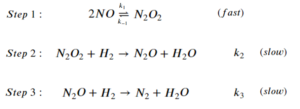Express reaction rate of the overall reaction using the steady-state approximation and the pre-equilibrium assumption. The expression should only include $[NO]$, $[H_2]$, and the rate constants of the elementary steps.

### Solution

Step 1: Set the sum of the rate of generation and consumption of intermediates to 0:

\begin{align*}
&\frac{d[N_2O_2]}{dt}=k_1[NO]^2-k_{-1}[N_2O_2]-k_2[N_2O_2][H_2]=0\;\;\;\;\;(1)\\
&\frac{d[N_2O]}{dt}=k_2[N_2O_2][H_2]-k_3[N_2O][H_2]=0\;\;\;\;\;(2)
\end{align*}

Step 2: Express the rate of the overall reaction:

$r=\frac{d[N_2]}{dt}=k_3[N_2O][H_2]$

Step 3: Replace the intermediate terms:

From (2):

$r=\frac{d[N_2]}{dt}=k_3[N_2O][H_2]=k_2[N_2O_2][H_2]$

Under pre-equilibria assumptions, we assume the rate of the equilibrium reaction is much faster than the reaction that consumes the product of the equilibrium reaction. So we can express $[N_2O_2]$ using the definition of equilibrium that the rate of forward and reverse reactions in step 1 are the same:
\begin{align*}
k_1[NO]^2&=k_{-1}[N_2O_2]\\
\frac{k_1[NO]^2}{k_{-1}}&=[N_2O_2]
\end{align*}

Step 4: Substitute the result of step 3 into the expression for r:

$r=k_2[N_2O_2][H_2]=\frac{k_2k_1[NO]^2[H_2]}{k_{-1}}$

The pre-equilibria assumption states that the rate of the equilibrium reaction is much faster than the reaction that consumes the product of the equilibrium reaction, which means $k_{-1}>>k_{2}$. Therefore, the $k_2[H_2]$ term is negligible comparing to the $k_{-1}$ term in the denominator under pre-equilibria assumption.

Reaction Mechanism Exercise 2

For the overall reaction to produce formaldehyde:

$H_2+CO \rightarrow H_2CO$

The step-wise elementary reactions are: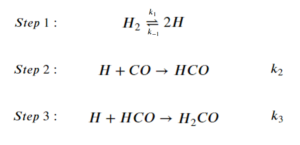Express reaction rate of the overall reaction using the steady-state approximation. The expression can involve the concentration of the reactants of the overall reaction, $[H]$, and the rate constants of the elementary steps. Then state how to replace $[H]$ in the expression (you do not have to calculate it).

### Solution

Step 1: Set the sum of the rate of generation and consumption of intermediates to 0. Notice how the coefficients affect the rate law:

\begin{align*}
&\frac{d[H]}{dt}=2k_1[H_2]-2k_{-1}[H]^2-k_2[H][CO]-k_3[H][HCO]=0\;\;\;\;\;(1)\\
&\frac{d[HCO]}{dt}=k_2[H][CO]-k_3[H][HCO]=0\;\;\;\;\;(2)
\end{align*}

Step 2: Express the rate of the overall reaction:

$r=\frac{d[H_2CO]}{dt}=k_3[H][HCO]$

Step 3: Replace the intermediate terms:

From (2):

$r=k_3[H][HCO]=k_2[H][CO]$

We can get an expression of $[H]$ by solving for it in equation (1), also using the relationship from (2) that $k_3[H][HCO]=k_2[H][CO]$:

\begin{align*}
2k_1[H_2]-2k_{-1}[H]^2-k_2[H][CO]-k_3[H][HCO]&=0\\
2k_1[H_2]-2k_{-1}[H]^2-2k_2[H][CO]&=0\\
\end{align*}

This is a quadratic equation about $[H]$. We can solve it using the quadratic formula:

$[H]=\frac{-b±\sqrt{b^2-4ac}}{2a}$

where $a = -2k_{-1}$, $b=-2k_2[CO]$, $c=2k_1[H_2]$

Reaction Mechanism Exercise 2 – with Pre-equilibria

For the overall reaction to produce formaldehyde:

$H_2+CO \rightarrow H_2CO$

The step-wise elementary reactions are: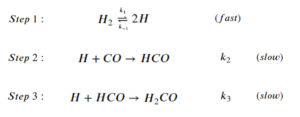Express reaction rate of the overall reaction using the steady-state approximation and the pre-equilibrium assumption. The expression should only include $[H_2]$, $[CO]$, and the rate constants of the elementary steps.

### Solution

Step 1: Set the sum of the rate of generation and consumption of intermediates to 0:

\begin{align*}
&\frac{d[H]}{dt}=2k_1[H_2]-2k_{-1}[H]^2-k_2[H][CO]-k_3[H][HCO]=0\;\;\;\;\;(1)\\
&\frac{d[HCO]}{dt}=k_2[H][CO]-k_3[H][HCO]=0\;\;\;\;\;(2)
\end{align*}

Step 2: Express the rate of the overall reaction:

$r=\frac{d[H_2CO]}{dt}=k_3[H][HCO]$

Step 3: Replace the intermediate terms:

From (2):

$r=k_3[H][HCO]=k_2[H][CO]$

Using the pre-equilibrium assumptions to replace [H]:
\begin{align*}
k_1[H_2]&=k_{-1}[H]^2\\
\frac{k_1}{k_{-1}}&=[H]^2\\
\Big(\frac{k_1}{k_{-1}}\Big)[H_2]^{\frac{1}{2}}&=[H]
\end{align*}

Step 4: Substitute the result of step 3 into the expression for r:

$r=k_2[H][CO]=k_2\Big(\frac{k_1}{k_{-1}}\Big)[H_2]^{\frac{1}{2}}[CO]$

Reaction Mechanism Exercise 3

For the reaction of ozone depletion:

$2O_3 \rightarrow 3O_2$

The step-wise elementary reactions are: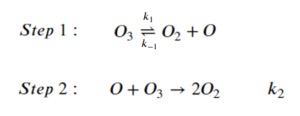Express reaction rate of oxygen gas generation $\big(\frac{d[O_2]}{dt}\big)$ using the steady-state approximation. The expression should include $[O_3]$, $[O_2]$, and the rate constants of the elementary steps.

### Solution

Step 1: Set the sum of the rate of generation and consumption of intermediates to 0:

$\frac{d[O]}{dt}=k_1[O_3]-k_{-1}[O_2][O]-k_2[O][O_3]=0\;\;\;\;\;(1)$

Step 2: Express $\frac{d[O_2]}{dt}$ from the elementary reactions. Notice that $O_2$ is generated in multiple elementary steps:

$\frac{d[O_2]}{dt}=k_1[O_3]-k_{-1}[O_2][O]+2k_{2}[O][O_{3}]$

Step 3: Replace $[O]$ using equation (1)

\begin{align*}
k_1[O_3]-k_{-1}[O_2][O]-k_2[O][O_3]&=0\\
k_1[O_3]&=k_{-1}[O_2][O]+k_2[O][O_3]\\
k_1[O_3]&=[O](k_{-1}[O_2]+k_2[O_3])\\
\frac{k_1[O_3]}{k_{-1}[O_2]+k_2[O_3]}&=[O]
\end{align*}

Step 4: Sub the expression for $[O]$ into $\frac{d[O_2]}{dt}$

\begin{align*}
\frac{d[O_2]}{dt}&=k_1[O_3]-k_{-1}[O_2][O]+2k_{2}[O][O_{3}]\\
\frac{d[O_2]}{dt}&=k_1[O_3]-\frac{k_{-1}k_1[O_3][O_2]}{k_{-1}[O_2]+k_2[O_3]}+\frac{2k_{2}k_1[O_3]^2}{k_{-1}[O_2]+k_2[O_3]}
\end{align*}

Reaction Mechanism Exercise 3 – with Pre-equilibria

For the reaction of ozone depletion:

$2O_3 \rightarrow 3O_2$

The step-wise elementary reactions are: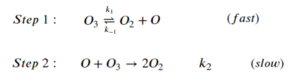Express reaction rate of oxygen gas generation $\big(\frac{d[O_2]}{dt}\big)$ using the steady-state approximation and the pre-equilibrium assumption.  The expression should include $[O_3]$, $[O_2]$, and the rate constants of the elementary steps.

### Solution

Step 1: Set the sum of the rate of generation and consumption of intermediates to 0:

$\frac{d[O]}{dt}=k_1[O_3]-k_{-1}[O_2][O]-k_2[O][O_3]=0$

Step 2: Express $\frac{d[O_2]}{dt}$:

$\frac{d[O_2]}{dt}=k_1[O_3]-k_{-1}[O_2][O]+2k_{2}[O][O_{3}]$

Step 3: Using the pre-equilibrium assumptions to replace [O]:

\begin{align*}
k_1[O_3]&=k_{-1}[O_2][O]\\
\frac{k_1[O_3]}{k_{-1}[O_2]}&=[O]
\end{align*}

Step 4: Sub the expression for $[O]$ into $\frac{d[O_2]}{dt}$

\begin{align*}
\frac{d[O_2]}{dt}&=k_1[O_3]-k_{-1}[O_2][O]+2k_{2}[O][O_{3}]\\
&=k_1[O_3]-k_{-1}[O_2]\frac{k_1[O_3]}{k_{-1}[O_2]}+\frac{2k_2k_1[O_3]^2}{k_{-1}[O_2]}\\
&=k_1[O_3]-k_1[O_3]+\frac{2k_2k_1[O_3]^2}{k_{-1}[O_2]}\\
&=\frac{2k_2k_1[O_3]^2}{k_{-1}[O_2]}\\
\end{align*}

## References

 Chemistry LibreTexts. 2020. 14.4: The Change Of Concentration With Time (Integrated Rate Laws). [online] Available at: <https://chem.libretexts.org/Bookshelves/General_Chemistry/Map%3A_Chemistry_-_The_Central_Science_(Brown_et_al.)/14%3A_Chemical_Kinetics/14.4%3A_The_Change_of_Concentration_with_Time_(Integrated_Rate_Laws)> [Accessed 23 April 2020].